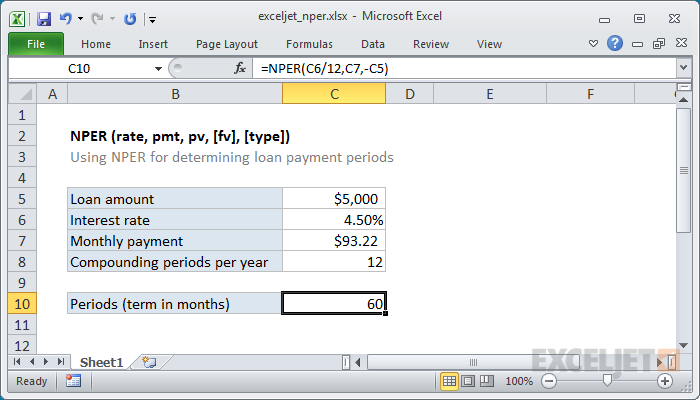Blog

# What Does Nper Mean in Excel?

Are you familiar with Microsoft Excel? It is an incredibly powerful spreadsheet software used by millions of people every day, and it contains a variety of handy features. One of these features is the NPER function, which is a financial formula used to calculate the number of periods for a loan or investment. If you’re curious about what NPER means in Excel and how it works, then you’ve come to the right place! In this article, we’ll discuss what NPER means in Excel and how you can use it to your advantage.# What does NPER Mean in Excel?

## Overview of NPER in Excel

NPER is a function in Excel that allows you to calculate the total number of periods in an annuity. It is commonly used to calculate loan payments and other time-based financial transactions. The NPER function is important to understand if you’re working with any type of loan or annuity.

NPER stands for “number of periods” and the function uses five parameters to calculate the total number of periods. The parameters include the rate of interest, the amount of payments, the present value of the annuity, the future value of the annuity, and whether the payments are made at the beginning or end of the period.

The NPER function is useful for financial professionals and anyone who needs to calculate loan payments or other types of annuities. This function makes it easy to calculate how long it will take to pay off a loan, or how much you’ll need to invest in order to reach a certain goal.

## How to Use NPER in Excel

Using the NPER function in Excel is straightforward. You’ll need to enter the five parameters into the function to get the total number of periods. The syntax for the function is: =NPER(rate, pmt, pv, fv, type).

The rate refers to the rate of interest for the annuity. The pmt refers to the amount of the payment for each period. The pv is the present value of the annuity. The fv is the future value of the annuity. Finally, the type refers to whether the payments are made at the beginning (type = 1) or end (type = 0) of the period.

For example, if you want to calculate the total number of payments for a loan with an interest rate of 6%, a payment of \$500 per month, and a present value of \$20,000, you would use the following formula: =NPER(6%, 500, 20000, 0, 0). This would give you a total of 60 payments.

## NPER vs. PMT in Excel

The NPER function is often confused with the PMT function in Excel. While both functions are related to loan payments, they are not the same. The PMT function is used to calculate the amount of each payment, while the NPER function is used to calculate the total number of periods.

To use the PMT function, you’ll need to enter the rate, number of payments, present value, and future value. The syntax for the function is: =PMT(rate, nper, pv, fv). For example, if you want to calculate the monthly payment for a loan with an interest rate of 6%, a total of 60 payments, and a present value of \$20,000, you would use the following formula: =PMT(6%, 60, 20000, 0). This would give you a payment of \$500 per month.

## Advantages of Using NPER in Excel

Using the NPER function in Excel can be a great way to save time and effort when calculating loan payments and other types of annuities. It eliminates the need to calculate each payment manually and makes it easy to get the total number of payments.

The NPER function is also useful for financial planning. It can help you determine how long it will take to pay off a loan or how much you’ll need to invest in order to reach a certain goal. This can help you make more informed decisions when it comes to investing and loan repayment.

## NPER in Other Spreadsheet Programs

The NPER function is available in most spreadsheet programs, including Google Sheets, Apple Numbers, and OpenOffice Calc. The syntax for the function may vary slightly, but the parameters are the same.

In Google Sheets, the NPER function is called “NPER” and the syntax is: =NPER(rate, pmt, pv, fv, type). For example, if you want to calculate the total number of payments for a loan with an interest rate of 6%, a payment of \$500 per month, and a present value of \$20,000, you would use the following formula: =NPER(6%, 500, 20000, 0, 0). This would give you a total of 60 payments.

### Apple Numbers

In Apple Numbers, the NPER function is called “NPV” and the syntax is: =NPV(rate, pmt, pv, fv, type). For example, if you want to calculate the total number of payments for a loan with an interest rate of 6%, a payment of \$500 per month, and a present value of \$20,000, you would use the following formula: =NPV(6%, 500, 20000, 0, 0). This would give you a total of 60 payments.

### OpenOffice Calc

In OpenOffice Calc, the NPER function is called “NPER” and the syntax is: =NPER(rate, pmt, pv, fv, type). For example, if you want to calculate the total number of payments for a loan with an interest rate of 6%, a payment of \$500 per month, and a present value of \$20,000, you would use the following formula: =NPER(6%, 500, 20000, 0, 0). This would give you a total of 60 payments.